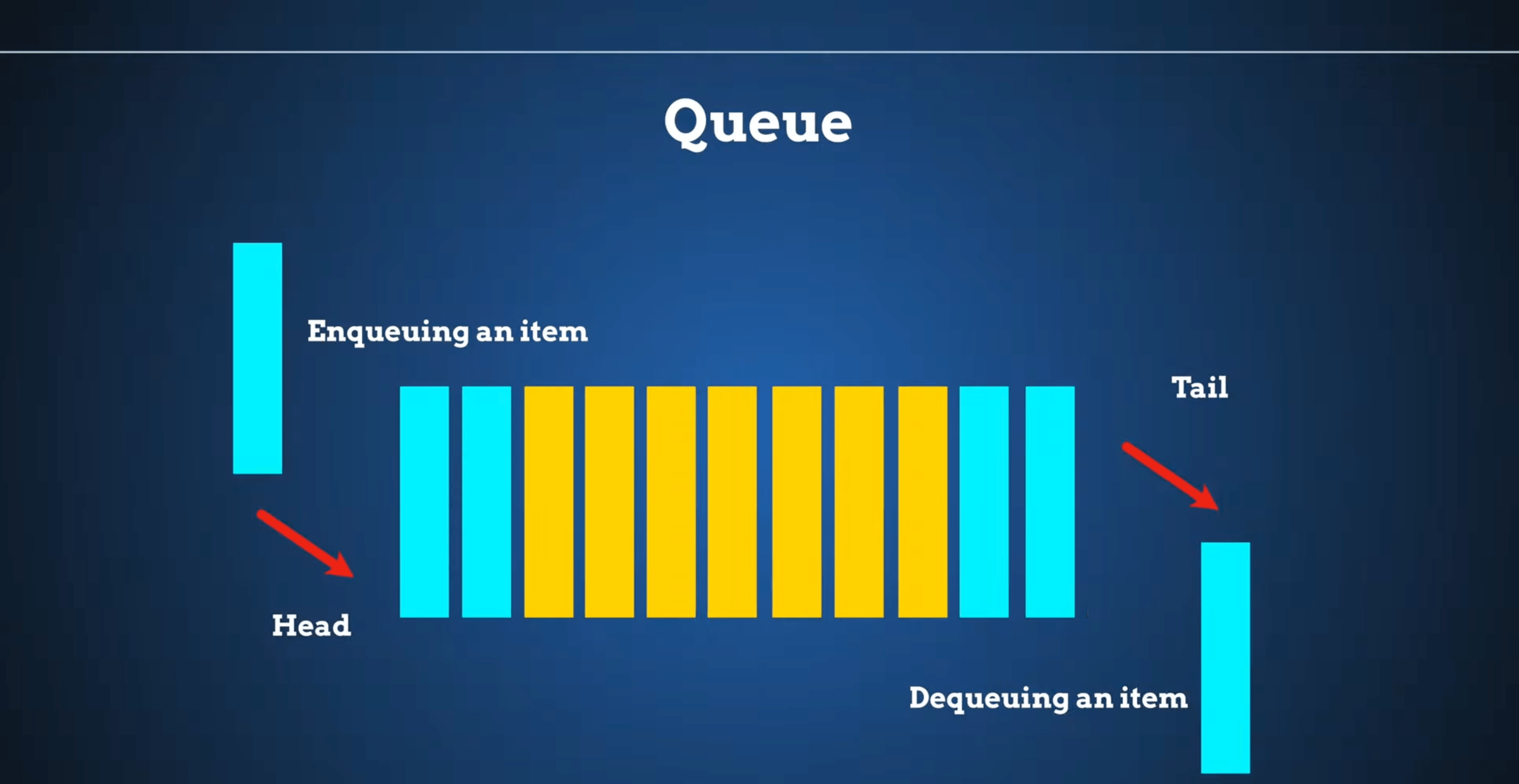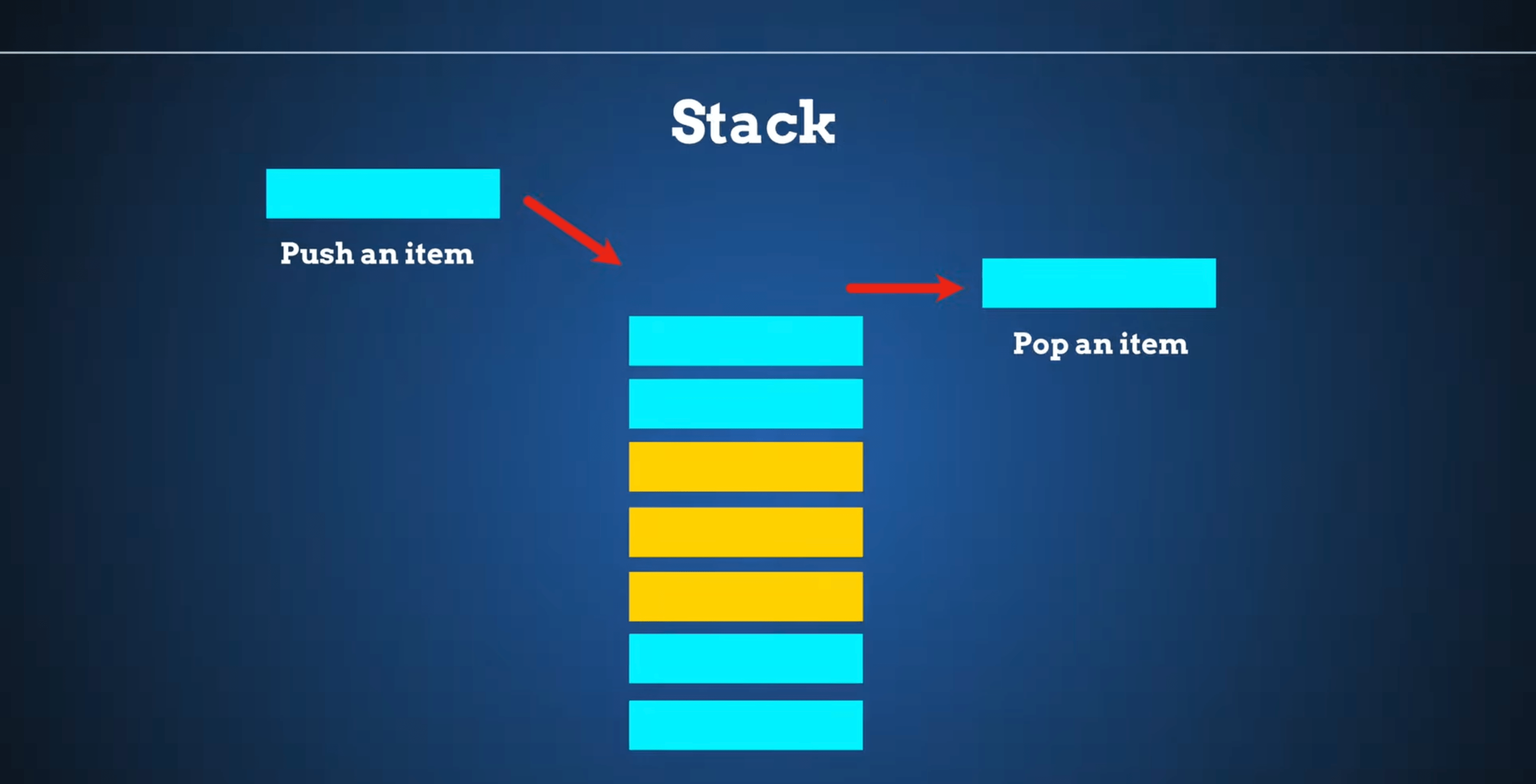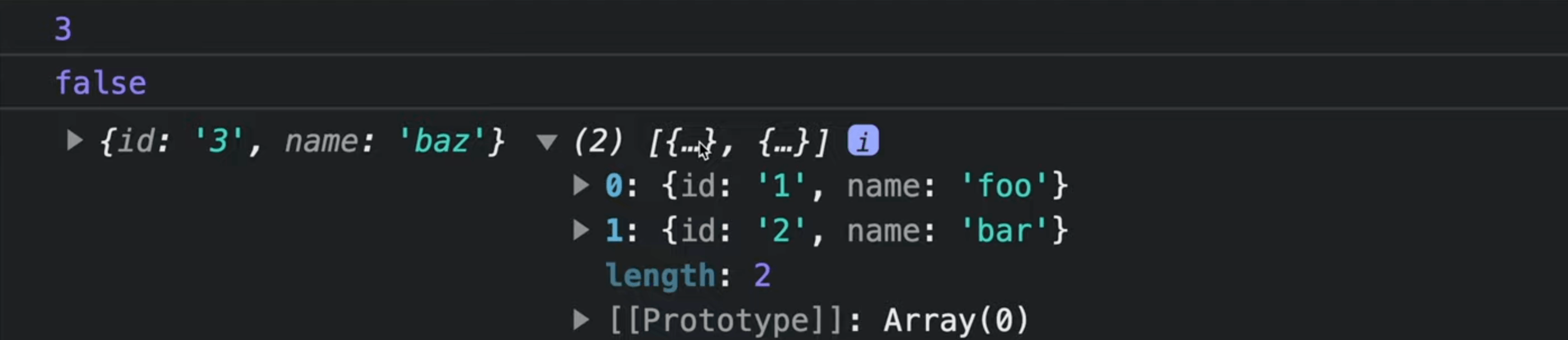Data Structure Stack with Javascript - Popular Interview Question
Data Structure Stack with Javascript - Popular Interview Question

In this post you will learn about such data structure as stack and we will implement it in Javascript.

## Stack & Queue

And actually this is a question that you can get on interviews really often. It is either "Please write the implementation of stack" or "What is the difference between stack and queue".

This is why first I want to answer on that specific question.

A stack follows a LIFO (Last In First Out) order, whereas a queue follows a FIFO (First in First Out) order for sorting elements.This is the diagram of queue data structureAnd this is the diagram of stack data structure.

So essentially these are similar data structures with this single difference.

## Stack implementation

Now let's implement Stack in plain Javascript. Actually it is much easier to implement Stack that Queue and it is really difficult to implement it wrong.

``````class Stack {
items = []

push(element) {
this.items.push(element)
}

pop() {
this.items.pop()
}
}
``````

We store elements in `items` array. To add elements in the array we use `push`. To implement `pop` method we just use `pop` function which removes the last added to the array element and returns it.

We must also implement several helper methods.

``````class Stack {
...
isEmpty() {
return this.size() === 0
}

size() {
return this.items.length
}

peek() {
return this.items[this.size() - 1]
}
}
``````

These are typical helper methods that we implement in the data structure. `size` returns the amount of elements in the array, `isEmpty` checks if we have elements at all and `peek` shows us the next element that will be removed.

Now let's create an instance of Stack and check some methods.

``````const stack = new Stack()
stack.push({id: '1', name: 'foo'})
stack.push({id: '2', name: 'bar'})
stack.push({id: '3', name: 'baz'})
console.log(stack.size())
console.log(stack.isEmpty())
const firstElement = stack.pop()
console.log(firstElement, stack.items)
``````Here we see the correct size, our stack is not empty and the element that we removed is the last element that we pushed. So we successfully implemented Stack data structure.

And actually if you want to improve your Javascript knowledge and prepare for the interview I highly recommend you to check my course Javascript Interview Questions.

📚 Source code of what we've done